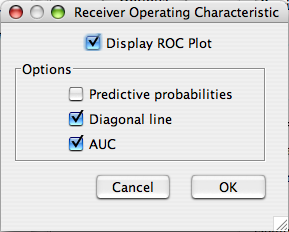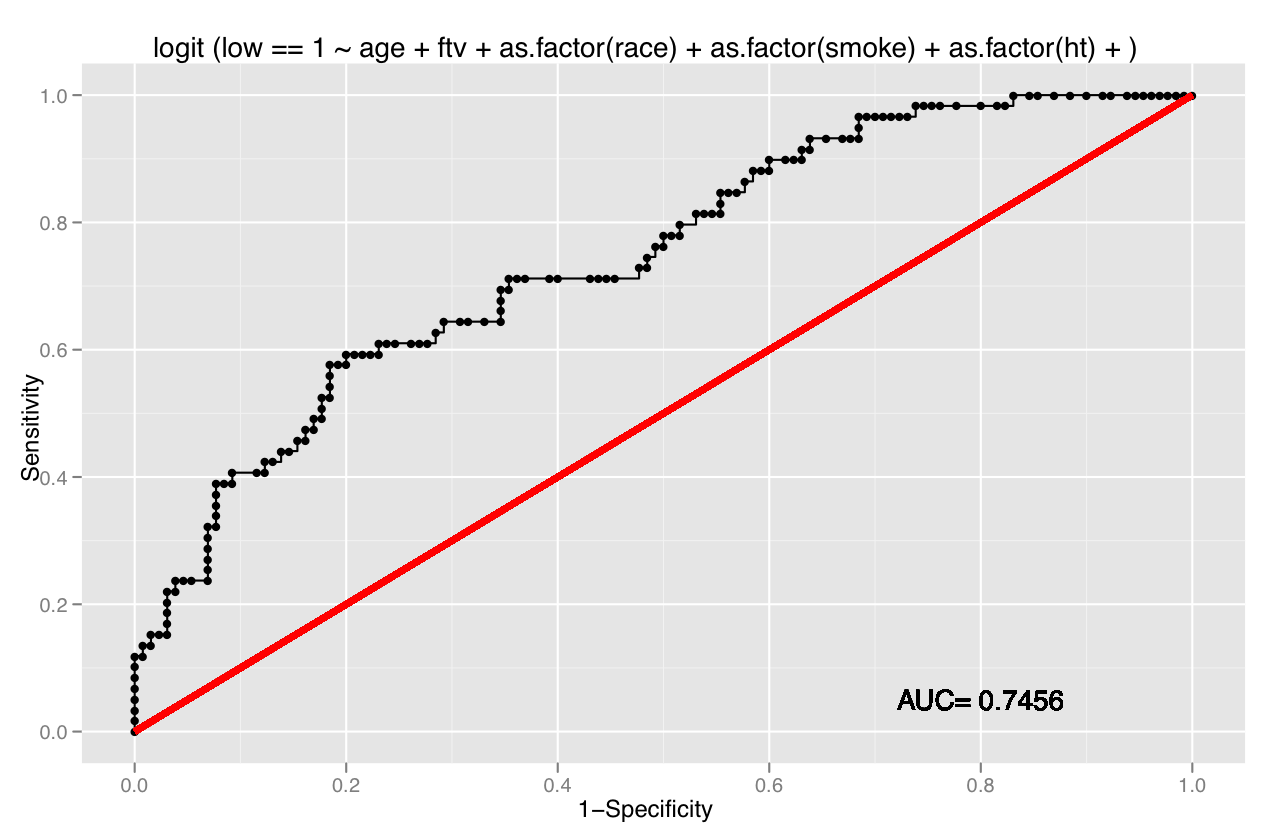The Receiver Operating Characteristic curve is a useful summary of how well the full logistic regression model functions as a predictor of outcome.

## Dialog• Predictive Probabilities displays the empirical probability of the model correctly classifying the data at each point on the ROC curve.
• Diagonal Line Displays a red diagonal line indicating the ROC curve associated with randomly guessing.
• AUC or Area Under the Curve is a single numerical measure of predictive power.